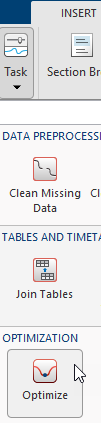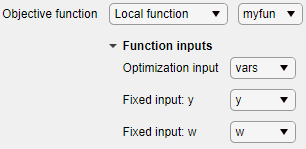# Optimize

Optimize in the Live Editor

## Description

The Optimize task lets you interactively optimize a nonlinear objective function or solve a nonlinear equation. The task automatically generates MATLAB® code for your live script.

• Specify the objective function, either by writing a function or browsing for a function.

• Specify solver options.

• Run the optimization.

If you have Optimization Toolbox™ or Global Optimization Toolbox you can solve more problem types using the Optimize task, such as solve a system of nonlinear equations or optimize an objective function with nonlinear constraints. For general information about Live Editor tasks, see Add Interactive Tasks to a Live Script.

To add the Optimize task to a live script in the MATLAB Editor, on the Live Editor Insert tab, select Task > Optimize.Alternatively, in a code block in the script, type a relevant keyword, such as `fminbnd`. Select Optimize from the suggested command completions.## Parameters

Optimization solver that MATLAB uses to solve the problem, specified as one of these solvers:

Example: `fminsearch`

Function to minimize (for `fminbnd` or `fminsearch`) or to find a root (for `fzero`), specified as a function handle, local function name, or function file.

• Function handle — Create the function handle in the workspace. Then, in the Optimize task, select Objective function > Function handle and choose the objective function.

• Local function

• New — In the Optimize task, select Objective function > Local function and then click the button. A new function definition appears below the task. Edit the function so that it calculates the objective. Then choose the local function.

• Existing — Select Objective function > Local function and choose the local function.

• Function file

• New — In the Optimize task, select Objective function > From file and then click the button. A new function file appears. Edit the file to calculate the objective, then save the file on the MATLAB path. Click the button and select the file.

• Existing — Choose `From file` then Browse... and select the file.

## Tips

• For functions with extra inputs, Optimize requires you to choose the optimization variable, and to specify which workspace variables contain the fixed data inputs. This example contains three function inputs:Optimize generates code only after you specify all function inputs.

• Optimize cannot parse a function containing the `varargin` input or a function that contains an error.

• If you select an objective function from a file, Optimize adds the file location to your MATLAB path.

• If Optimize has a parsing error or if multiple local functions have the same name, the list of available local functions is empty.

## Version History

Introduced in R2020b